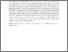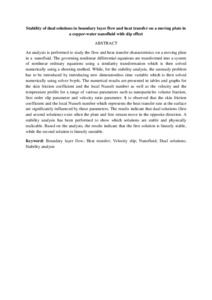# Stability of dual solutions in boundary layer flow and heat transfer on a moving plate in a copper-water nanofluid with slip effect

## Citation

Bachok @ Lati, Norfifah and Najib, Najwa and Md. Arifin, Norihan and Senu, Norazak (2016) Stability of dual solutions in boundary layer flow and heat transfer on a moving plate in a copper-water nanofluid with slip effect. WSEAS Transantions on Fluid Mechanics, 11 (19). pp. 151-158. ISSN 1790-5087; ESSN: 2224-347X

## Abstract

An analysis is performed to study the flow and heat transfer characteristics on a moving plate in a nanofluid. The governing nonlinear differential equations are transformed into a system of nonlinear ordinary equations using a similarity transformation which is then solved numerically using a shooting method. While, for the stability analysis, the unsteady problem has to be introduced by introducing new dimensionless time variable which is then solved numerically using solver bvp4c. The numerical results are presented in tables and graphs for the skin friction coefficient and the local Nusselt number as well as the velocity and the temperature profile for a range of various parameters such as nanoparticles volume fraction, first order slip parameter and velocity ratio parameter. It is observed that the skin friction coefficient and the local Nusselt number which represents the heat transfer rate at the surface are significantly influenced by these parameters. The results indicate that dual solutions (first and second solutions) exist when the plate and free stream move in the opposite direction. A stability analysis has been performed to show which solutions are stable and physically realizable. Based on the analysis, the results indicate that the first solution is linearly stable, while the second solution is linearly unstable.Preview
Text
Stability of dual solutions in boundary layer flow and heat transfer .pdfView Item